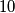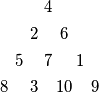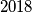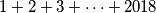### IMO Shortlist 2018 problem C4

Kvaliteta:
Avg: 0.0
Težina:
Avg: 7.0

An anti-Pascal triangle is an equilateral triangular array of numbers such that, except for the numbers in the bottom row, each number is the absolute value of the difference of the two numbers immediately below it. For example, the following is an anti-Pascal triangle with four rows which contains every integer from$1$ to$10$.Does there exist an anti-Pascal triangle with$2018$ rows which contains every integer from$1$ to$1 + 2 + 3 + \dots + 2018$?

Source: https://www.imo-official.org/problems/IMO2018SL.pdf